### Introduction to Earth Data Analysis

1. Get Started
1. Learn Git/Github for team collaboration
2. Install Python via Anaconda
3. Install Python IDE (Jupyter Notebook, Visual Studio Code)
4. Python variables and data types
5. Install and use Python packages
6. Get familiar with Numpy for data manipulations
7. Get familiar with Matplotlib for plotting arrays
8. Get familiar with Pandas to analyze tabular data
2. Get familiar with text file formats
1. Use basic Markdown syntax to format text in Jupyter Notebook files
2. Get familiar with text file formats - CSV, .txt, YAML
3. Read/Write data from text files using Python
4. Read/Write data from text files using Pandas
5. Deal with missing data in Pandas
3. Spatial Data Analysis
1. Read multi-layered raster data (.tif / .hdf / .nc) in Python
2. Read vector data (shapefiles) using geopandas
3. GIS in Python
4. Time-series Analysis using Pandas
2. Work with `Datetime` formats
3. Resample time series
4. Filtering/smoothing time-series with Pandas
5. Plot time series
5. How to plot topographic high-resolution geospatial maps

## Recent posts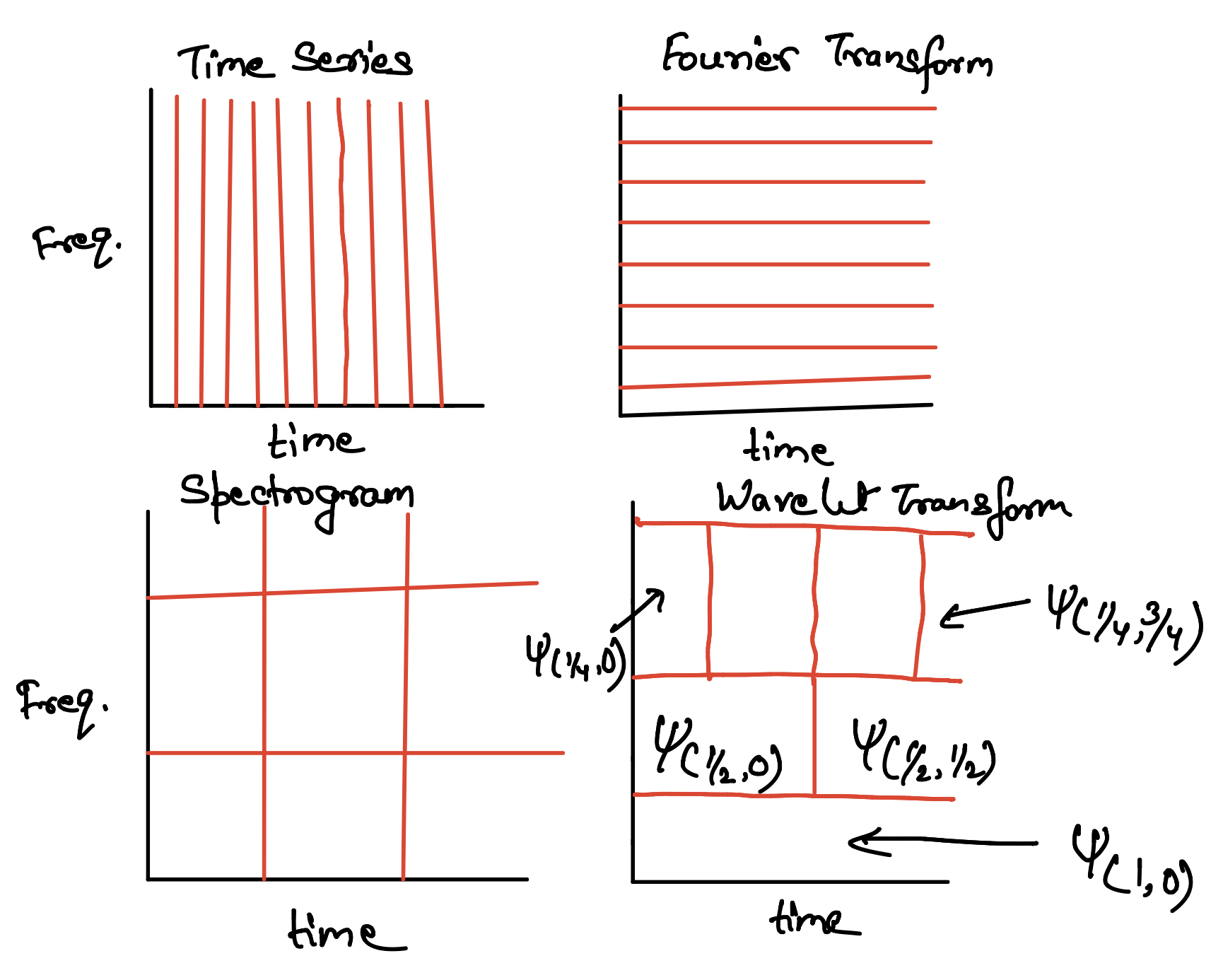## Towards multi-resolution analysis with wavelet transform

We will learn the basic concepts of wavelet tranform and multi-resolution analysis starting from the Fourier Transform, and Gabor Transform.## Empirical orthogonal function analysis to inspect the spatial coherency in the geospatial data (codes included)

Empirical Orthogonal Functions analysis decomposes the continuous space-time field into a set of orthogonal spatial patterns along with a set of associated u...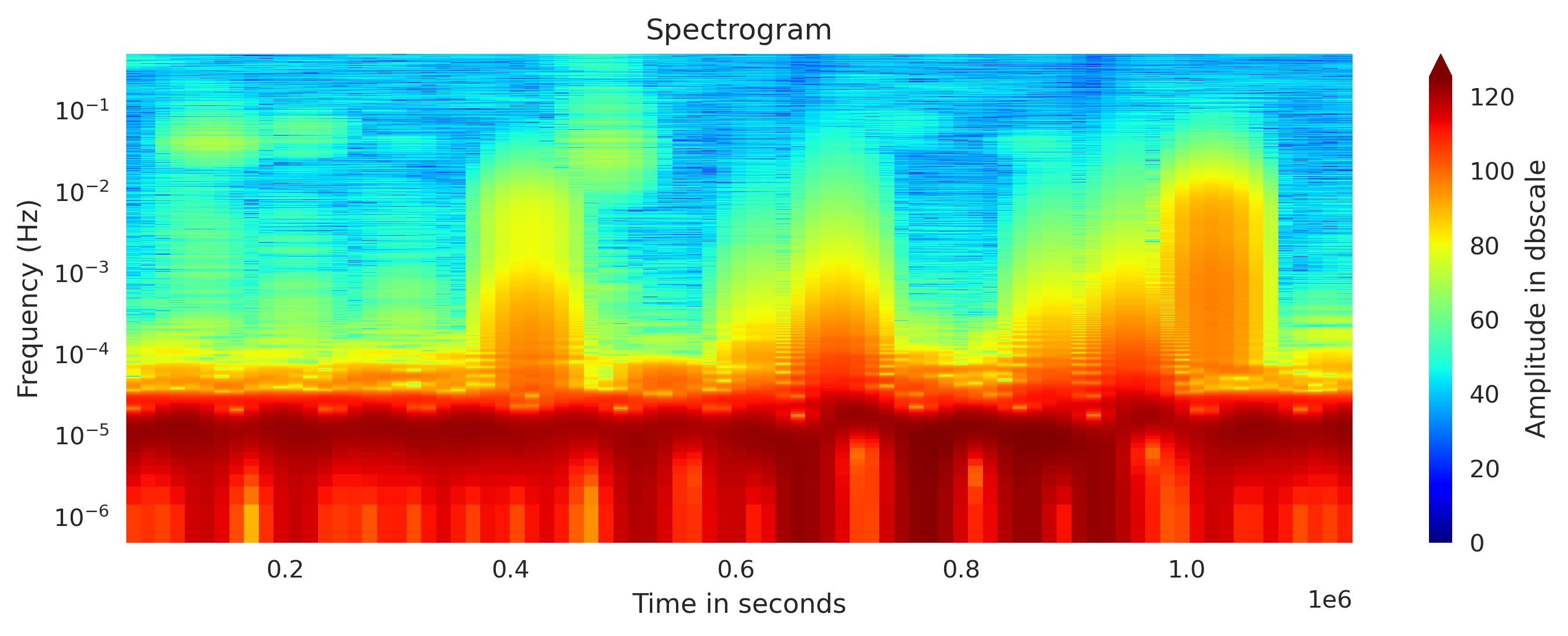## Concatenating daily seismic traces into one miniseed file (codes included)

I concatenate the daily seismic traces for 15 days into one miniseed file for further analysis. Then I obtained the spectrogram of the 15 days seismic data. ...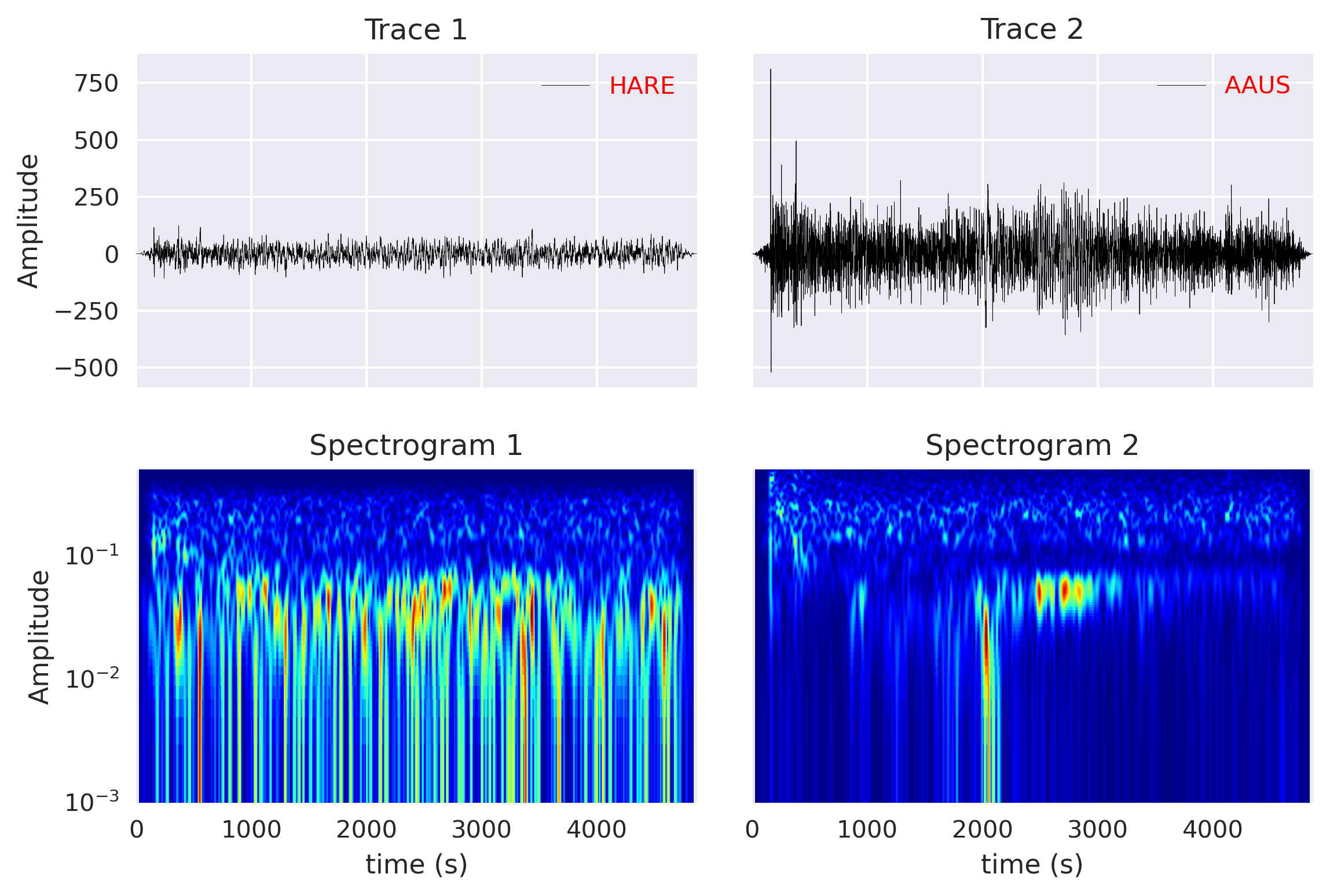## Computing cross-correlation and spectrogram of two seismic traces (codes included)

Read the seismic traces from the miniseed files and compute the cross-correlation and spectrogram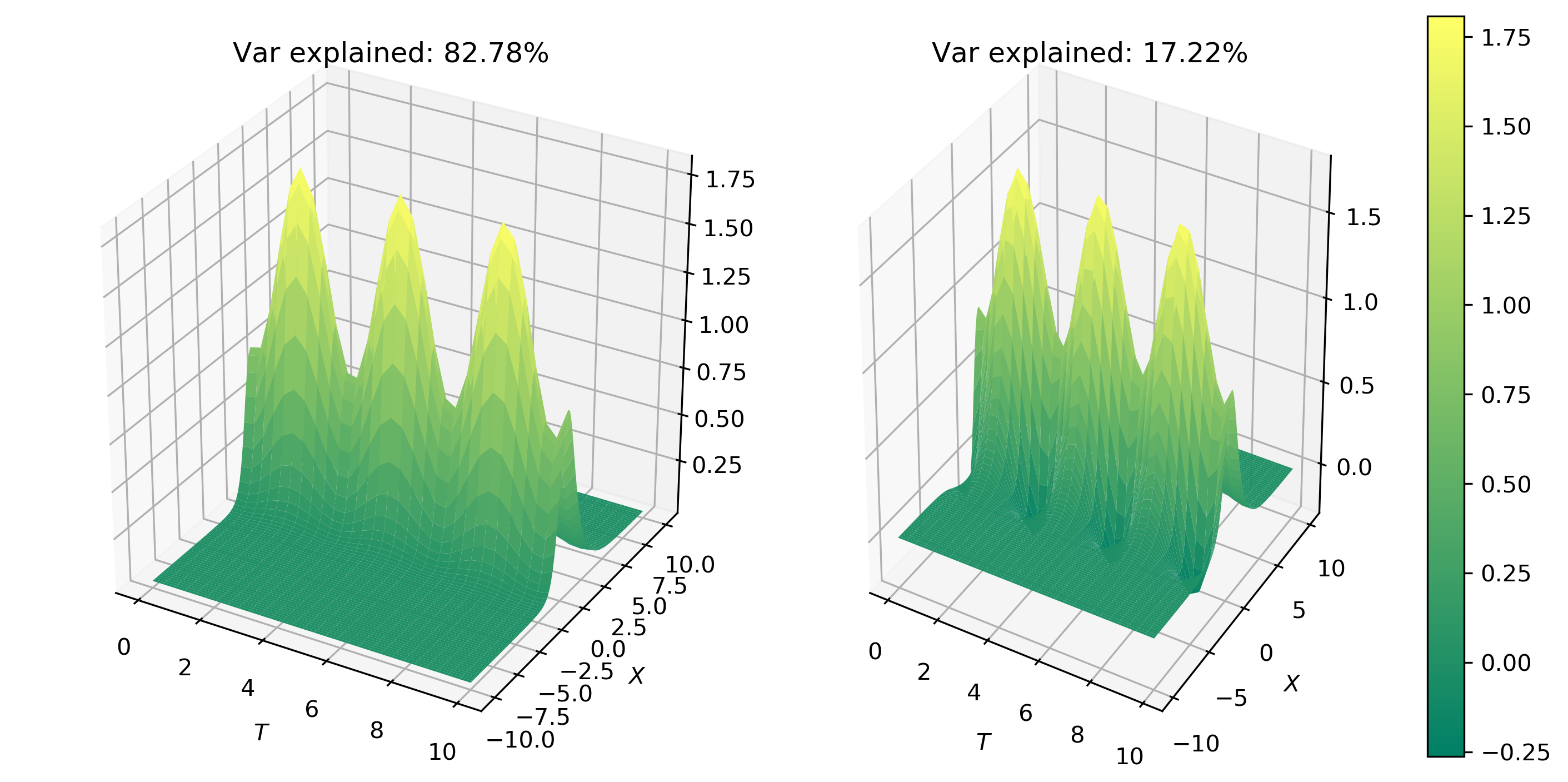## Principal component analysis to decompose signals and reduce dimensionality (codes included)

We will learn the basics of Fourier analysis and implement it to remove noise from the synthetic and real signals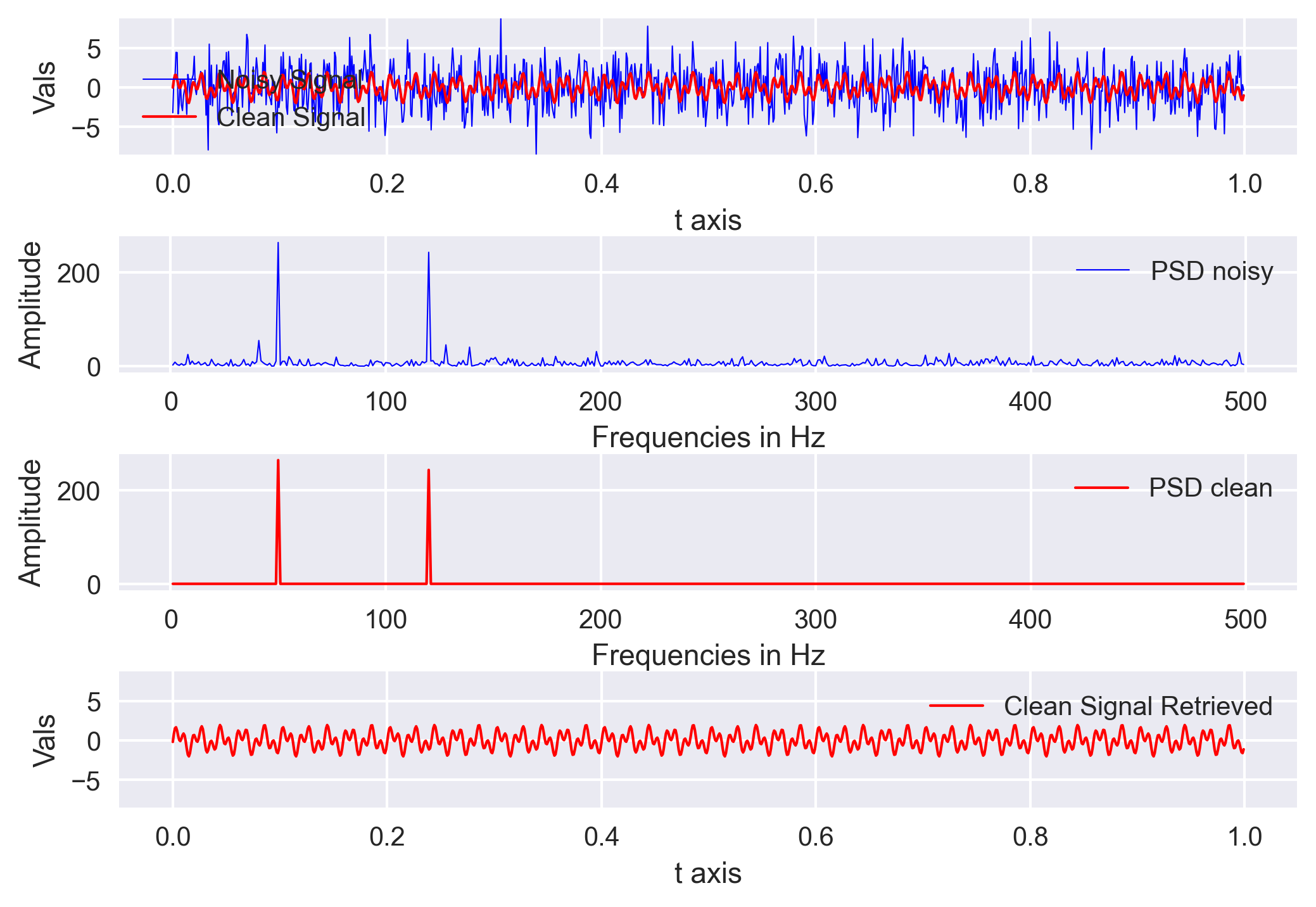## Signal denoising using fourier analysis in python (codes included)

We will learn the basics of Fourier analysis and implement it to remove noise from the synthetic and real signals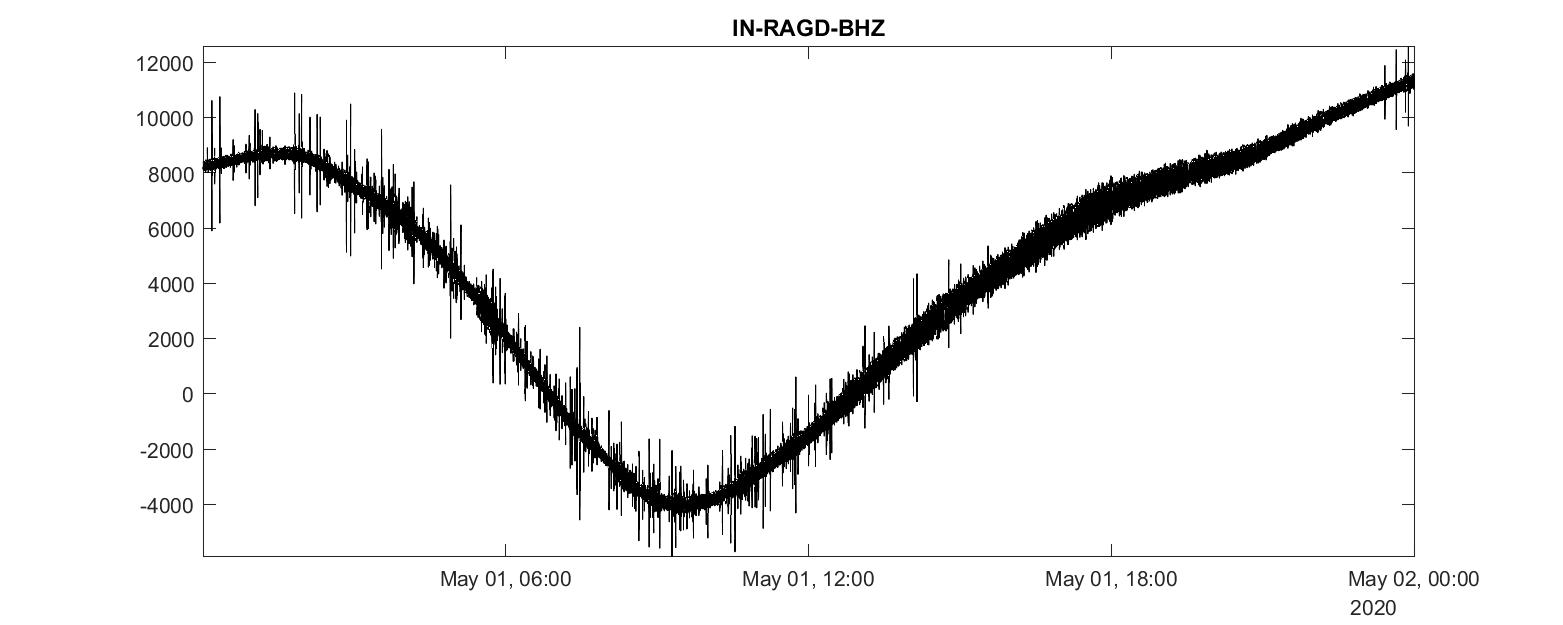## Analyzing miniseed seismic data in matlab (codes included)

We will learn how to convert a mseed data file into mat format and then read and analyze it using MATLAB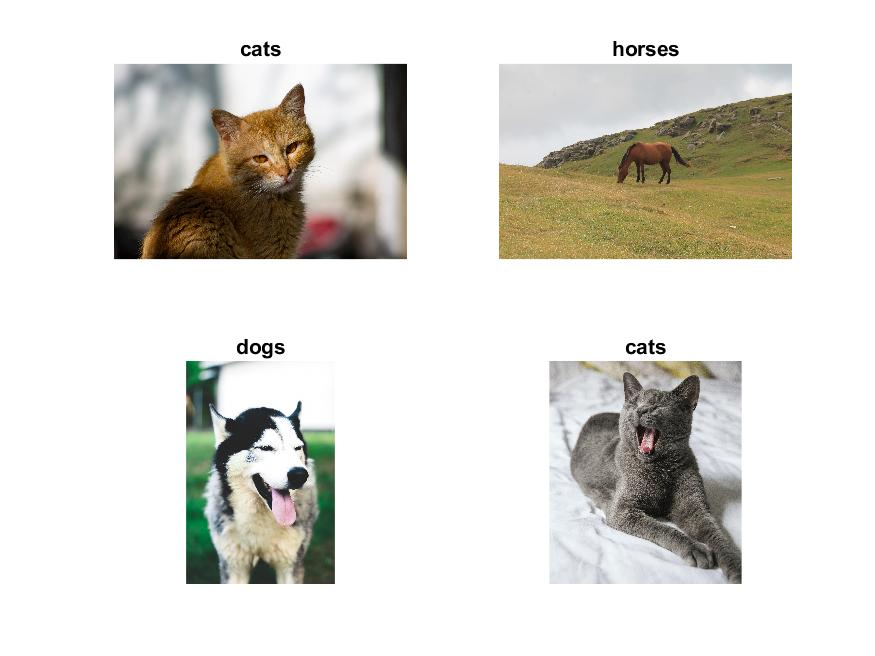## Transfer learning applied on the unsplash data using alexnet pretrained network (codes included)

Transfer learning using the pre-trained deep learning networks from MATLAB can be easily implemented to achieve fast and impressive results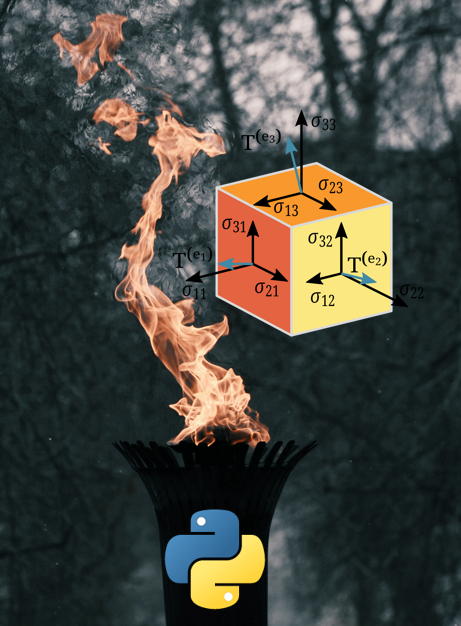## Getting familiar with pytorch data structures (codes included)

In this introduction to the concepts of Pytorch data structures, we will learn about how to create and reshape tensors using Pytorch and compare it with the ...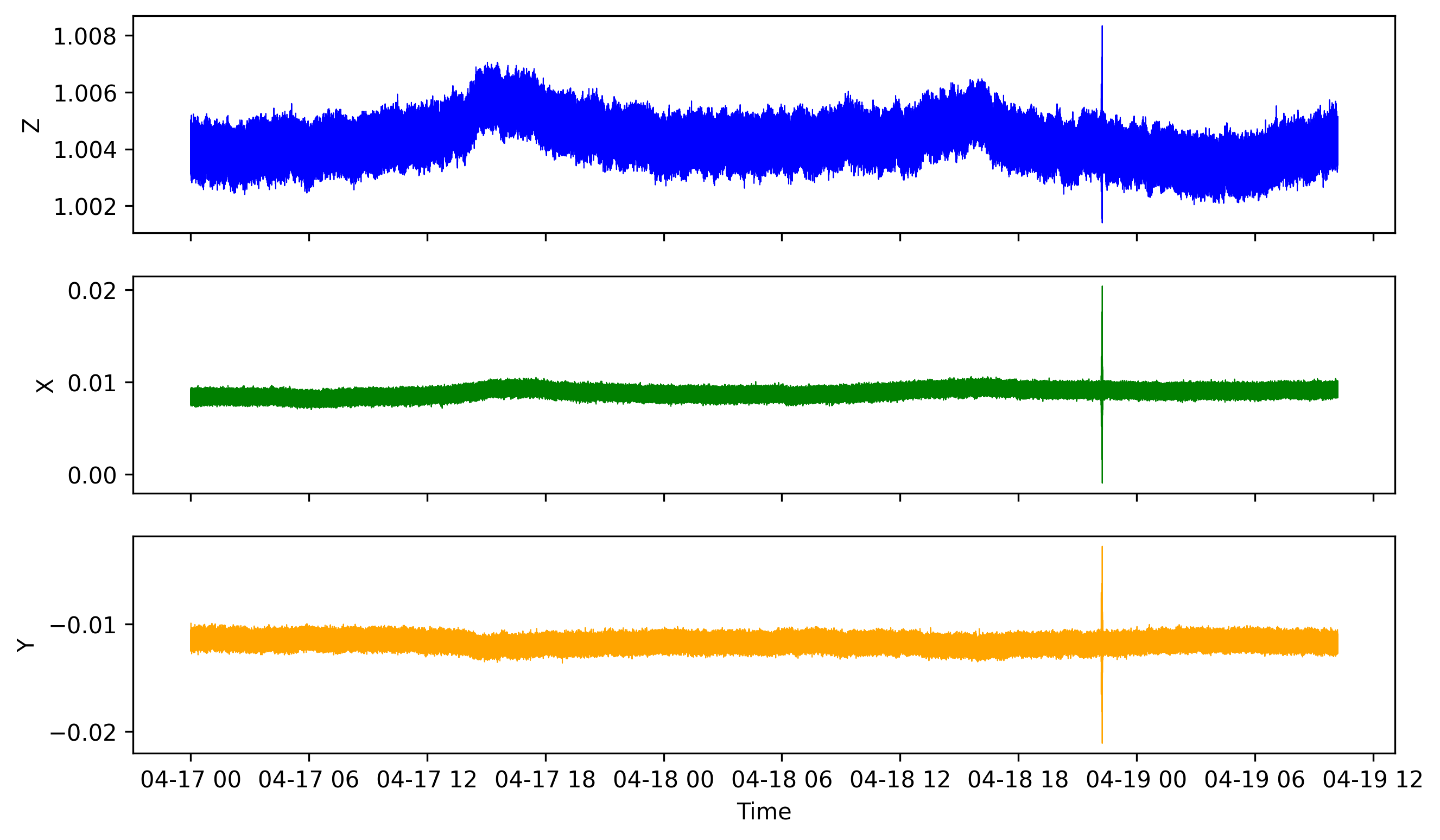## How to analyze a huge data file with pandas (codes included)

We learn how to read huge csv file containing time series data by breaking it into chunks and then visualizing it with matplotlib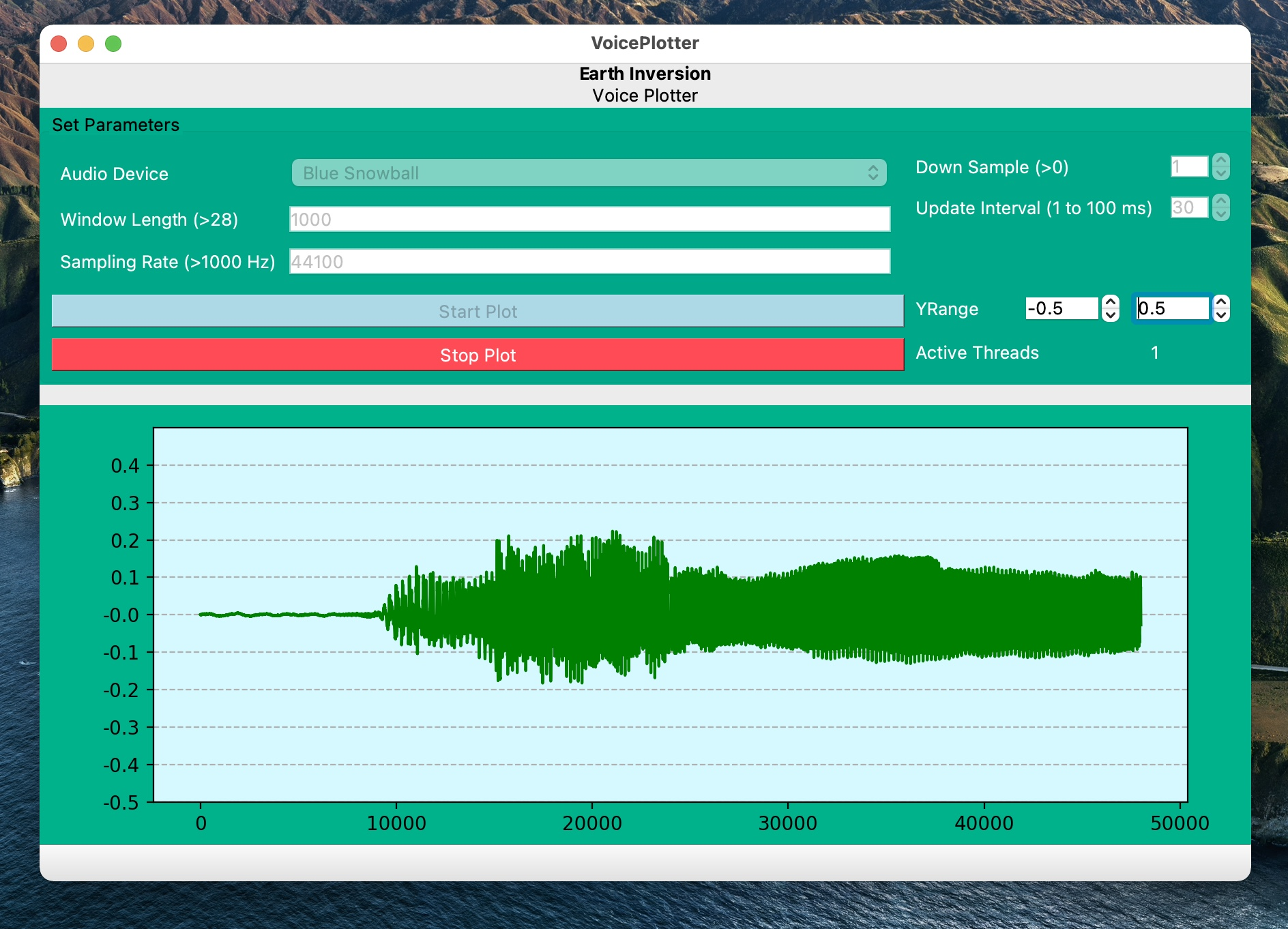## Pyqt5 application for visualizing ambient sound in real-time (codes included)

A PyQt5 application for retrieving and visualizing sound waveforms in real time. Codes included.## How to start using pandas immediately for earth data analysis (codes included)

This tutorial gives a brief description of scientific computing using Pandas by introducing Series, DataFrame, Pandas common operations, methods, conditional...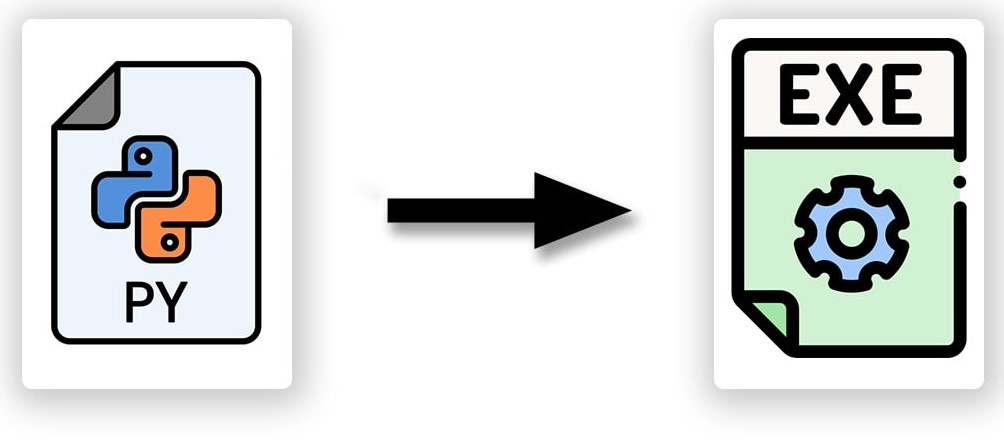## Make your python script executable from anywhere in linux

Follow the instructions to make your python script executable from anywhere in Linux system.## How to securely send data from local client to remote server using paramiko (codes included)

Paramiko module can be used in Python to securely send data from the local client to the remote server. It is analogous to the SSH and SCP in Linux.## Towards multi-resolution analysis with wavelet transform

We will learn the basic concepts of wavelet tranform and multi-resolution analysis starting from the Fourier Transform, and Gabor Transform.## Empirical orthogonal function analysis to inspect the spatial coherency in the geospatial data (codes included)

Empirical Orthogonal Functions analysis decomposes the continuous space-time field into a set of orthogonal spatial patterns along with a set of associated u...## Concatenating daily seismic traces into one miniseed file (codes included)

I concatenate the daily seismic traces for 15 days into one miniseed file for further analysis. Then I obtained the spectrogram of the 15 days seismic data. ...## Computing cross-correlation and spectrogram of two seismic traces (codes included)

Read the seismic traces from the miniseed files and compute the cross-correlation and spectrogram## Principal component analysis to decompose signals and reduce dimensionality (codes included)

We will learn the basics of Fourier analysis and implement it to remove noise from the synthetic and real signals## Signal denoising using fourier analysis in python (codes included)

We will learn the basics of Fourier analysis and implement it to remove noise from the synthetic and real signals## Analyzing miniseed seismic data in matlab (codes included)

We will learn how to convert a mseed data file into mat format and then read and analyze it using MATLAB## Transfer learning applied on the unsplash data using alexnet pretrained network (codes included)

Transfer learning using the pre-trained deep learning networks from MATLAB can be easily implemented to achieve fast and impressive results## Getting familiar with pytorch data structures (codes included)

In this introduction to the concepts of Pytorch data structures, we will learn about how to create and reshape tensors using Pytorch and compare it with the ...## How to analyze a huge data file with pandas (codes included)

We learn how to read huge csv file containing time series data by breaking it into chunks and then visualizing it with matplotlib## Pyqt5 application for visualizing ambient sound in real-time (codes included)